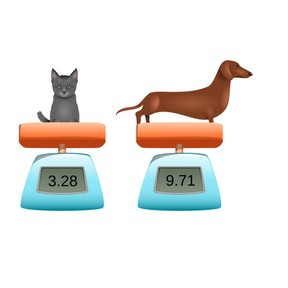Estimated and exact sum with decimal numbers with 1 and 2 decimal places

# Estimated and exact sum with decimal numbers with 1 and 2 decimal places

No account needed.8,000 schools use Gynzy92,000 teachers use Gynzy1,600,000 students use Gynzy

## General

Students learn to add decimal numbers with 1 and 2 decimal places and to estimate the sum and calculate the exact sum.

5.NBT.B.7

## Relevance

It is useful to be able to estimate and give the exact sum of decimal numbers with 1 and 2 decimal places so you can check if your exact answer is correct by using the estimated total. You need this to calculate about how much something weighs in total.

## Introduction

The interactive whiteboard shows three decimal numbers. Students must determine which of the three decimal numbers is the largest number. They can write the letter A, B, or C. Emphasize that a number with two decimal places is not always larger than a number with one decimal place.

## Development

Check that students are able to add decimal numbers with 1 and 2 decimal places by asking the following questions:
- How do you round decimal numbers if you want to estimate a sum?
- How do you add decimal numbers if you want to determine the exact sum?
- What is useful to do when adding decimal numbers with 1 decimal place to decimal numbers with 2 decimal places?

## Guided practice

Students first practice adding decimal numbers with 1 and 2 decimal places. Then students are asked to calculate using a story problem.

## Closing

Discuss with students that estimating the sum is useful because it gives an indication of what the exact sum should be- providing you a check for your exact total, as they should be quite close to each other. Check that students are able to calculate the estimated and exact sum by adding a 0 to the end of a decimal number and by splitting the second addend or both addends. As a class, solve the addition problem with weights given on the interactive whiteboard.

## Teaching tips

If students have difficulty with this learning goal, they can use the number line to add. Encourage them to take smaller jumps in their addition to help support their learning.

### The online teaching platform for interactive whiteboards and displays in schools

• Save time building lessons

• Manage the classroom more efficiently

• Increase student engagement